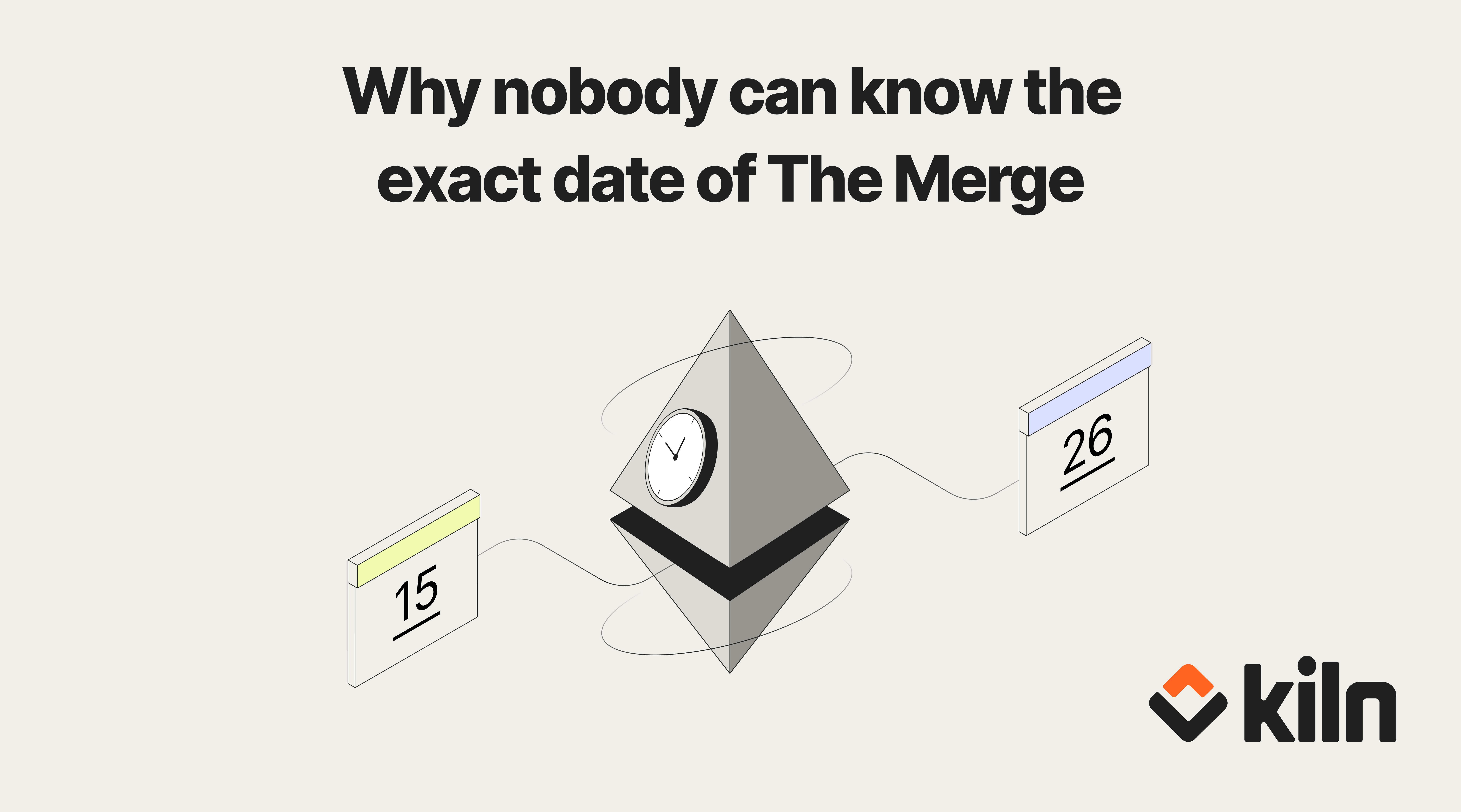Emmanuel Nalepa
Engineering
August 22, 2022

# Why nobody can know the exact date of The Merge

The Ethereum Merge will mark the end of proof-of-work for Ethereum, and the full transition to proof-of-stake. It will reduce Ethereum's energy consumption by ~99.95%.

The target date is September 15, 2022, but this estimate might have even a week of error. This blog post will explain why the famous "Wen Merge?" question cannot be answered with a definitive date.

## Usual protocol upgrades

Usually, protocols upgrades (like Istanbul, Berlin and London) come into effect at a given block height.

For example, on the mainnet, the Istanbul upgrade was effective at block 9,069,000. This block height is known in advance. Because we also know the average block time (~13 sec on Ethereum), we can determine quite precisely in advance the date of the given upgrade.

For example, if:

• current block height is 9,000,000
• current date is January 1st 2:00 PM
• Istanbul upgrade happens at block 9,069,000
• average block time is ~13 sec

We can deduce that the upgrade will happen in (9,069,000 - 9,000,000) * 13 sec = 897,000 sec = 10 days 9 hours 10 minutes.

==> The Istanbul upgrade will happen on January 11th 11:10 PM.

## The Merge protocol upgrade

Contrary to usual protocol upgrades, The Merge upgrade is not based on a block height, but on a Terminal Total Difficulty (also called TTD). For Ethereum mainnet, the TTD has been set to the following value: 58,750,000,000,000,000,000,000.

Now, let's explain what Difficulty, Total Difficulty & Terminal Total Difficulty are.

### Difficulty

In a pre-merge, proof-of-work world, miners have to solve a cryptographic puzzle in order to add a new block on the tip of the blockchain. The difficulty of this puzzle is regularly adjusted so a new block is added every ~12 seconds.

If the total mining computational power (also called hashrate) suddenly doubles, then a new block will be found every ~6sec. In order to compensate that, the difficulty will eventually double to recover a ~12 seconds block time.

On the contrary, if the total mining computational power is suddenly divided by a factor 2,  then a new block will be found every ~24sec. In order to compensate that, the difficulty will eventually be divided by a factor 2 to recover a ~12 seconds block time.

==> The difficulty is a number which can increase or decrease, depending on the total mining computational power (among other factors).

For example the difficulty of block 42 was 17,416,275,986

### Total difficulty

The total difficulty of a given block is simply the sum of the difficulty of all blocks from the genesis block to the given block.

For example:

This also can be seen recursively, where:

Total difficulty of block <n> = Total difficulty of block <n-1> + Difficulty of block <n>

Because difficulty is a positive number, the total difficulty can only increase over the time.

For example the total difficulty of block 42 is 741,927,801,599

For example the difficulty of block 43 is 17,424,780,027

We get the result: Total difficulty of block 43 = Total difficulty of block 42 + Difficulty of block 43.

## Terminal Total difficulty (or TTD)

Terminal total difficulty is the target value of the total difficulty where The Merge will happen.

As said previously, for Ethereum Mainnet, the TTD has been set to 58,750,000,000,000,000,000,000.

It means that, when a block has a total difficulty higher or equal to this value, then the transition from proof-of-work to proof-of-stake will happen.

While we cannot know the exact time when the total difficulty reaches the terminal total difficulty, we can estimate it. We know the current difficulty, so we can extrapolate the future values of total difficulty. It leads us to an estimation date for The Merge.

If the total mining computational power stays the same (which seems to be the case so far!), then The Merge will happen on Sep 15 12:00 2022 UTC.

However, if at a point in time, the total mining computational power doubles, then the remaining time before The Merge will be divided by 2. (And if the total mining computational power is divided by 2, then the remaining time before The Merge will double.)

## What is the reason for having a protocol upgrade based on TTD instead of a classical block height?

The rationale for this choice is explained in EIP-3675:

Using a pre-defined block number for the hardfork is unsafe in this context due to the PoS fork choice taking priority during the transition.

An attacker may use a minority of hash power to build a malicious chain fork that would satisfy the block height requirement. Then the first PoS block may be maliciously proposed on top of the PoW block from this adversarial fork, becoming the head and subverting the security of the transition.

To protect the network from this attack scenario, difficulty accumulated by the chain (total difficulty) is used to trigger the upgrade.

Here's to a successful Merge at TDD 58,750,000,000,000,000,000,000!

Thanks @manunalepa